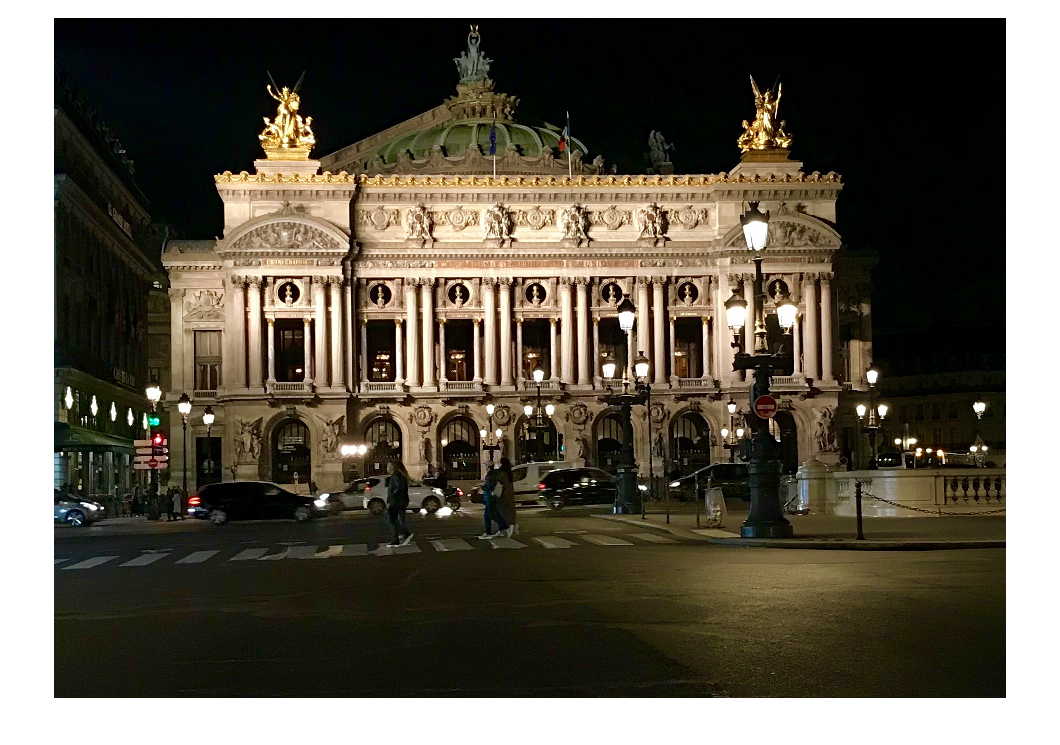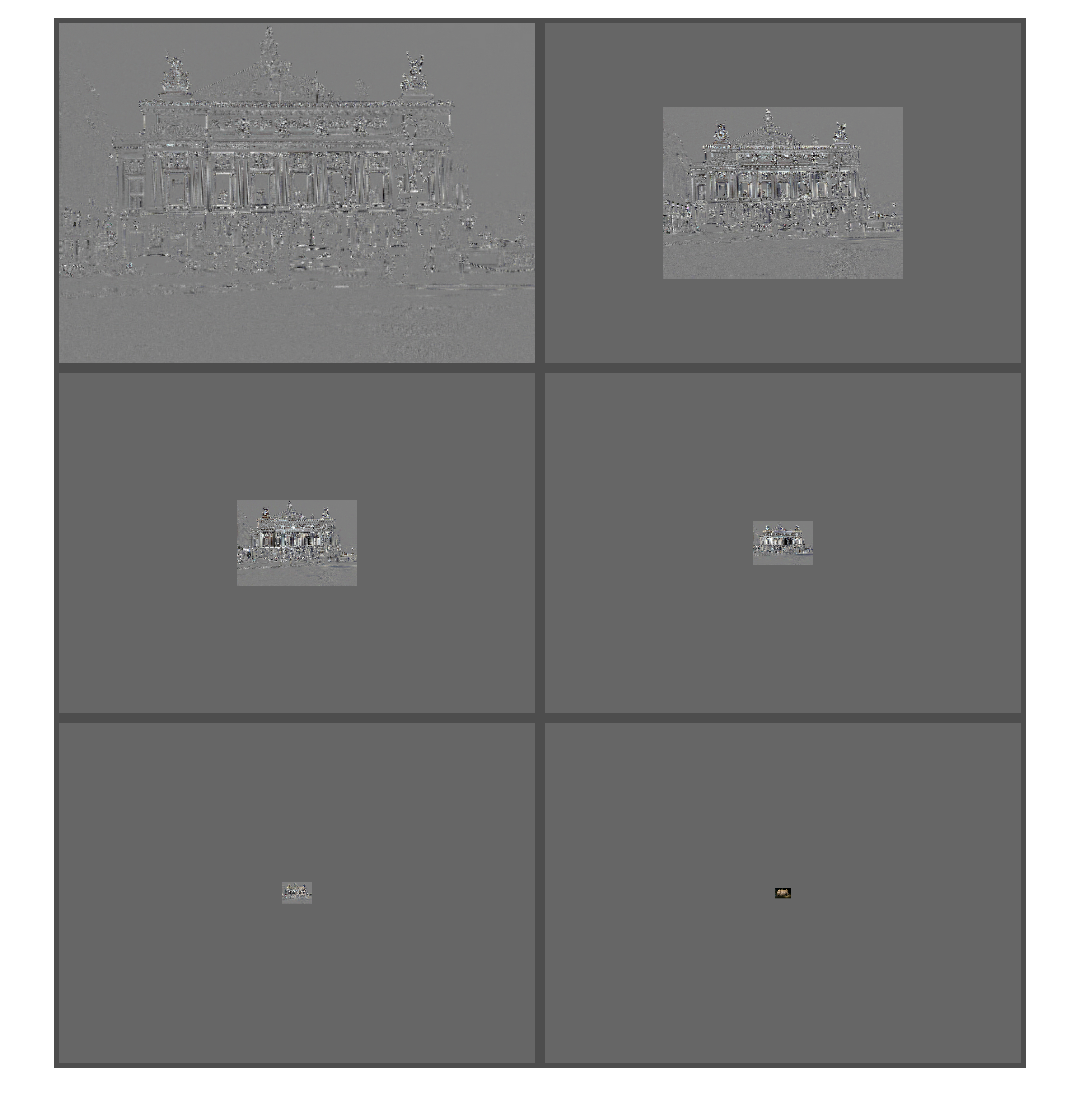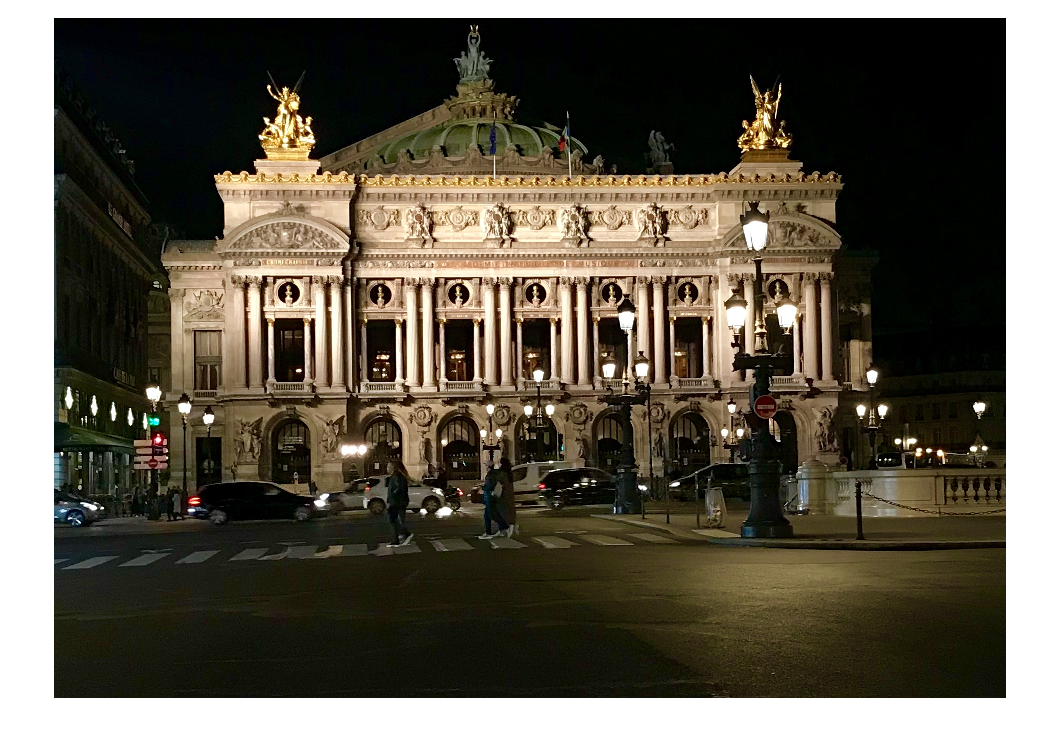# Multiresolution pyramids part 3: Laplacian pyramids

In my April 2 post, I introduced multiresolution pyramids, and I explained my dissatisfaction with the function impyramid, which (sadly) I designed. (Aside: I had that post ready to go on April 1, but I decided that maybe I should wait a day to publish it.) I followed that up on April 9 with an alternative computation method and interface for computing such a pyramid.

Today, I'll introduce a variation called the Laplacian pyramid. While the ordinary multiresolution pyramid contains a bunch of images that have been successively lowpass filtered and downsampled, the Laplacian pyramid contains a bunch of images that have essentially been bandpass filtered and downsampled. Burt and Adelson described the Laplacian pyramid as a data structure useful for image compression in "The Laplacian Pyramid as a Compact Image Code," IEEE Transactions on Communications, vol. COM-31, no. 4, April 1983, pp. 532-540.

The i-th image in the Laplacian pyramid is computed by taking the (i+1)-st image in the multiresolution pyramid, expanding it by a factor of 2, and subtracting the result from the i-th multiresolution pyramid image. The only thing to be particulary careful about is to handle the case where the level i image is not exactly twice the size of the level (i+1) image. If you compute the multiresolution pyramid the way I did last time, that can only happen between the first two levels.

Let's try it on the image I used last time.

A = imread('https://blogs.mathworks.com/steve/files/IMG_9968.jpg');
imshow(A)First, we need to compute the multiresolution pyramid using the function I presented previously.

mrp = multiresolutionPyramid(im2double(A));


Now we follow the computational recipe that I described in words above.

lapp = cell(size(mrp));
num_levels = numel(mrp);
lapp{num_levels} = mrp{num_levels};
for k = 1:(num_levels - 1)
A = mrp{k};
B = imresize(mrp{k+1},2,'lanczos3');
[M,N,~] = size(A);
lapp{k} = A - B(1:M,1:N,:);
end
lapp{end} = mrp{end};


Another function, showLaplacianPyramid (also at the end of this script), visualizes the pyramid.

showLaplacianPyramid(lapp)Given a Laplacian pyramid, we can reconstruct the original image by reversing the recipe above.

num_levels = numel(lapp);
B = lapp{end};
for k = (num_levels - 1) : -1 : 1
B = imresize(B,2,'lanczos3');
Lk = lapp{k};
[M,N,~] = size(Lk);
B = B(1:M,1:N,:) + Lk;
end
imshow(B)See the function reconstructFromLaplacianPyramid below.

In the Burt and Adelson paper, a key idea was to quantize the level images in the Laplacian pyramid so that they could be stored using fewer bits per pixel (on average). To simulate the effect, I'll round each pixel in the Laplacian level images (except the last one) to the nearest 0.1 (in a normalized range) and reconstruct from that.

for k = 1:(numel(lapp) - 1)
lapp_quantized{k} = round(lapp{k},1);
end
lapp_quantized{numel(lapp)} = lapp{end};

B_coded = reconstructFromLaplacianPyramid(lapp_quantized);
imshow(B_coded)You can see the distortions resulting from this crude quantization method, but the overall image is surprisingly recognizable and even detailed considering how much information we tossed away.

Next time, I plan to discuss the application that I was originally interested when I started writing this series on multiresolution pyramids: image blending.

function lapp = laplacianPyramid(mrp)

% Steve Eddins
% MathWorks

lapp = cell(size(mrp));
num_levels = numel(mrp);
lapp{num_levels} = mrp{num_levels};
for k = 1:(num_levels - 1)
A = mrp{k};
B = imresize(mrp{k+1},2,'lanczos3');
[M,N,~] = size(A);
lapp{k} = A - B(1:M,1:N,:);
end
lapp{end} = mrp{end};
end

function showLaplacianPyramid(p)

% Steve Eddins
% MathWorks

M = size(p{1},1);
N = size(p{1},2);

stretch_factor = 3;

for k = 1:numel(p)
Mk = size(p{k},1);
Nk = size(p{k},2);

if (k < numel(p))
else
end
A = p{k};
p{k} = A;
end

for k = 1:(numel(p)-1)
p{k} = (stretch_factor*p{k} + 0.5);
end

imshow(imtile(p,'GridSize',[NaN 2],...
'BorderSize',20,'BackgroundColor',[0.3 0.3 0.3]))
end

function out = reconstructFromLaplacianPyramid(lapp)

% Steve Eddins
% MathWorks

num_levels = numel(lapp);
out = lapp{end};
for k = (num_levels - 1) : -1 : 1
out = imresize(out,2,'lanczos3');
g = lapp{k};
[M,N,~] = size(g);
out = out(1:M,1:N,:) + g;
end
end


Published with MATLAB® R2019a

|